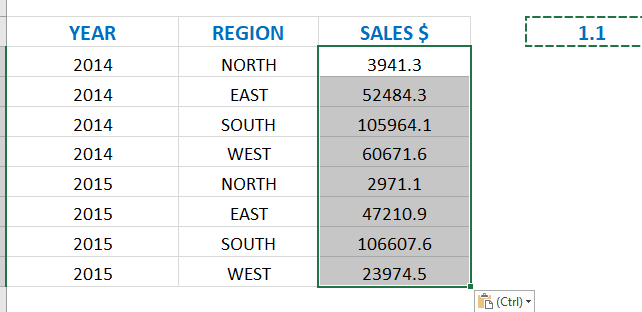Paste Special has a few different Paste features and operations that many users do not know about.

One of them is the Paste Special > Values > Multiply combination.

What that does is multiply a range of selected cells by a value, called the multiplier.  So if you want to increase all your values by 10%, you can….see how below:

STEP 1: Enter the amount to multiply by (the multiplier) in an empty cell, e.g. 1.10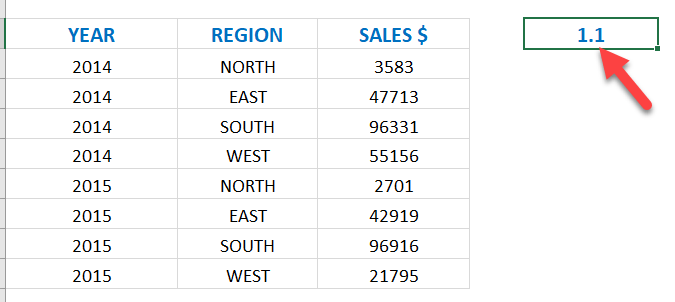STEP 2: Copy that cell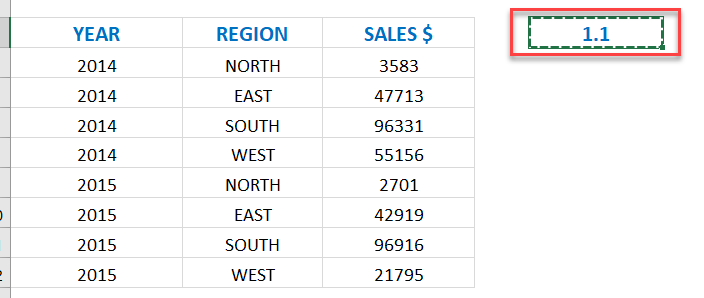STEP 3: Select the data range you want to multiply, Right Click and select Paste Special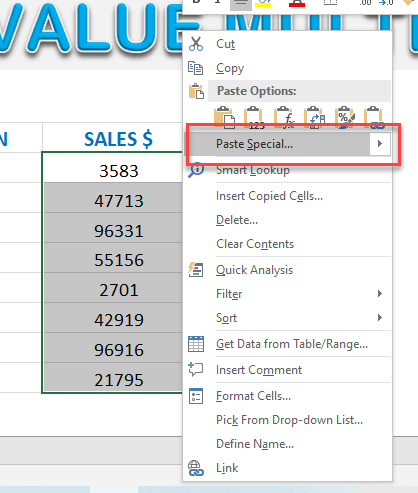STEP 4: Select Values & Multiply and press OK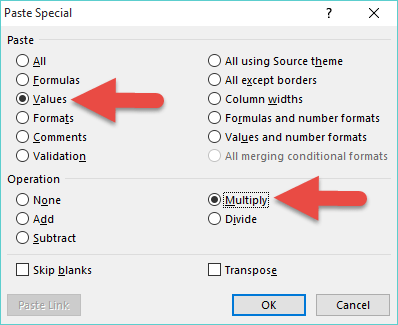Your values have now been multiplied by the multiplier!